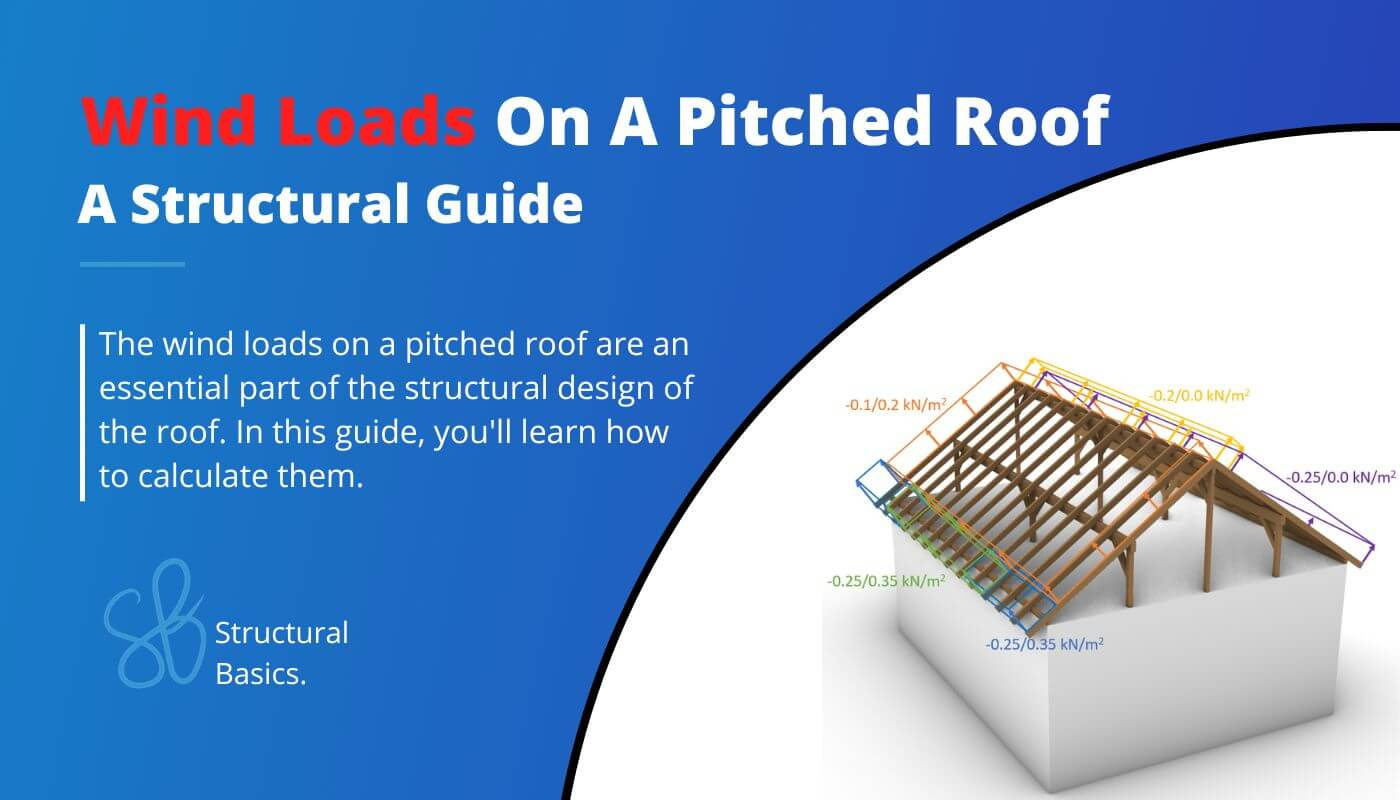# Wind Loads On A Pitched Roof {A Structural Guide}As in the previous articles, we are calculating the wind load according to EN 1991-1-4:2005. This time: The wind load of pitched roofs, as it is the case for most family houses.

In this article, we will calculate the wind loads for the example of a simple purlin roof. ❗But the static system does not influence the wind load, only the slope (angle of rafters).

The following picture shows only the structural elements of the roof. The ground floor which is also subjected to wind is simplified with a grey box.

This article builds up on the peak velocity pressure, which we calculated in a previous article for a precast office building.

We are not repeating the calculation in this article, but define a value $q_p$ for it. The calculation steps of the wind load of the walls is the same as for the precast concrete building.

Just keep in mind that the values differ due to different parameters such as location and geometry.

In order to calculate the wind load or wind pressure on external surfaces of a pitched roof, we are going to do the following steps:

1. Calculate the wind velocity pressure $q_{p}$
2. Define the outer geometry of the building
3. Calculate the width of the Wind areas
4. Find the external pressure coefficients

## Geometry and parameters from wind velocity pressure

This is a quick summary of the values we calculate to get the wind velocity pressure.

## Wind pressure on surfaces

### Wind pressure on external surfaces $w_{e}$

Now we have written the exact same words and formulas already in the articles about the wind load of the flat roof and walls, but isn’t it good to repeat things, so they stick better with us? 🤔

Anyway the formula (EN 1991-1-4:2005 (5.1)) to calculate the wind pressure on external surfaces is

$w_{e} = q_{p} \cdot c_{pe}$

Where

The coefficient $c_{pe}$ has 2 different values depending on the wind loaded area. There is a value for a surface area of 1 $m^2$ and 10 $m^2$. These two values can also be written as

Now, we already explained it a bit more detailed here. If you want to a more detailed explanation then either go to the article or read up in EN 1991-1-4:2005 7.2.

EN 1991-1-4 Table 7.3a and Table 7.3b give recommendations for $c_{pe.10}$ and $c_{pe.1}$.

❗This means that you have to double-check with your National annex because those values might be defined differently there❗

Tables 7.3a and 7.3b give values for 4 different areas F, G, H and I of our roof. Those areas depend on where the wind comes from and the shape of the roof.

Eurocode differentiates between mono- and duo pitched roofs.

A mono pitched roof has basically only “one slope”. So no change in slope.

The duo pitch roof has basically “two slopes” which change at the highest point of the roof.

For “our” duo pitched roof, we can define the Areas for both wind directions – Wind from the front and from the side.

We will be talking about wind from front and side in this article. The following picture emphasizes what we mean by front and side.

### Wind from Front

For the case that the wind blows from the front direction, we can define some geometric dimensions of the building according to EN 1991-1-4:2005

From those dimensions we can define $e$ which determines the widths and depths of Areas F, G, H, J and I according to EN 1991-1-4:2005 Figure 7.8

$e = min(b, 2h)$

$e = min(13.0m, 2*6.0m=12.0m) = 12.0m$

From e we get the dimensions of the areas according to EN 1991-1-4:2005 Figure 7.8

So many numbers – I know. Let’s visualize them 😁

Okay, now let’s go back to the formula for the wind pressure for external surfaces and derive the values. The peak velocity pressure was calculated as

$q_{p} = 0.5 \frac{kN}{m^2}$

The external pressure coefficients for duopitch roofs with wind from front (direction angle $\Theta$) can be taken from EN 1991-1-4:2005 Table 7.4a.

For a roof slope angle of 29° $\approx$ 30° we get

Based on our coefficients, we can now calculate the Wind pressure on external surfaces.

When you calculate the wind loads the first time ever, it might be very confusing in which direction you have to apply the loads. So let’s apply the wind loads with the $c_{pe.10}$ coefficient (for 10 $m^2$) on our building.

Now we also have to do the same for the case that wind comes from the side.

### Wind from Side

In the scenario that wind comes from the side, we have to define the Area widths again. We have to redefine the geometry parameters.

From those dimensions we can define $e$ which determines the widths and depths of Areas F, G, H and I according to EN 1991-1-4:2005 Figure 7.8

$e = min(b, 2h)$

$e = min(9.0m, 2*6.0m=12.0m) = 9.0m$

From e we get the dimensions of the areas according to EN 1991-1-4:2005 Figure 7.8

As we all know, a picture tells more than a thousand words – so let’s visualize all of those numbers 😁

The external pressure coefficients for duo pitched roofs with the wind direction of $\Theta$ = 90° and a roof slope angle of 29° $\approx$ 30° can be taken from EN 1991-1-4:2005 Table 7.4b

Based on our coefficients, we can now calculate the Wind pressure on external surfaces.

Those wind area loads we can now visualize.